# PC controller via root locusThis Homework Help Question: "PC controller via root locus" No answers yet.

We need 10 more requests to produce the answer to this homework help question. Share with your friends to get the answer faster!

0 /10 have requested the answer to this homework help question.

Once 10 people have made a request, the answer to this question will be available in 1-2 days.
All students who have requested the answer will be notified once they are available.

#### Earn Coin

Coins can be redeemed for fabulous gifts.

Similar Homework Help Questions
• ### PD controller design via root locus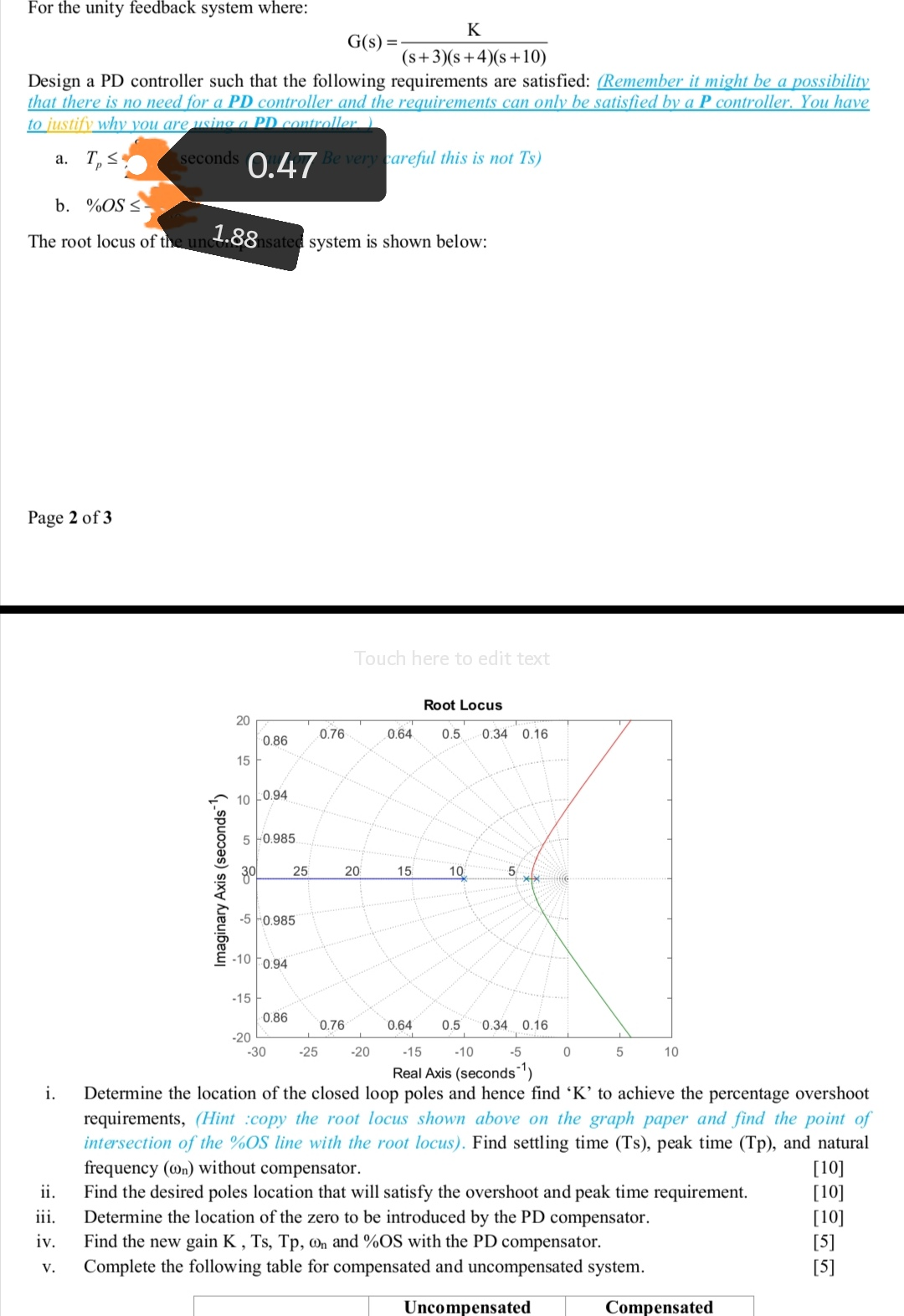• ### b) Design a PID controller via root-locus to satisfy the following requirements for the controlled system...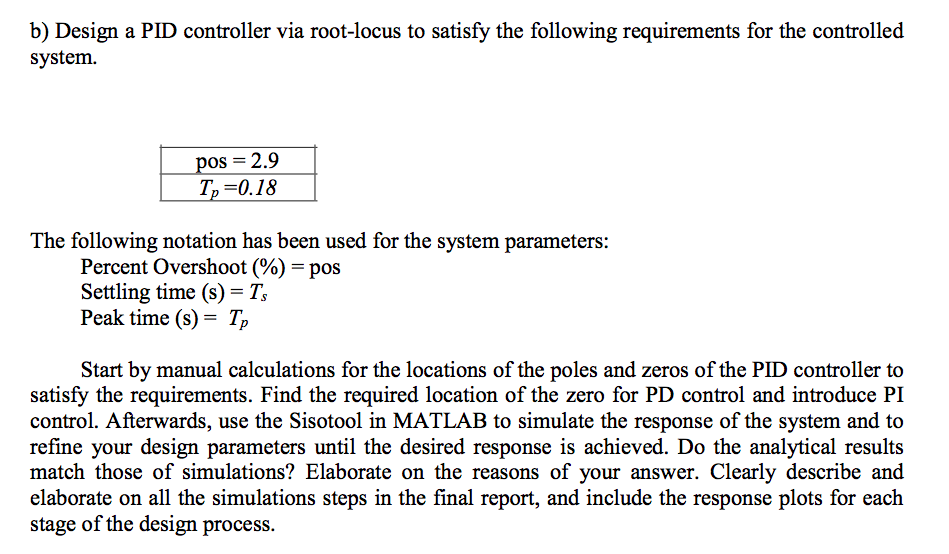b) Design a PID controller via root-locus to satisfy the following requirements for the controlled system 2.9 T,-0.18 The following notation has been used for the system parameters: Percent Overshoot(%)-pos Settling time (s) Peak time (s)- Tp Start by manual calculations for the locations of the poles and zeros of the PID controller to satisfy the requirements. Find the required location of the zero for PD control and introduce PI control. Afterwards, use the Sisotool in MATLAB to simulate the...

• ### Problem 2: Sketching a root locus plot The bank angle controller for an airplane is given...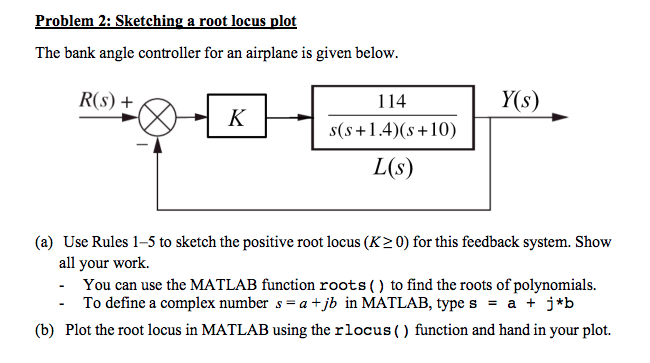Problem 2: Sketching a root locus plot The bank angle controller for an airplane is given below. R(s) + (+14(s+10) L(s) (a) Use Rules 1-5 to sketch the positive root locus (K20) for this feedback system. Show all your work. You can use the MATLAB function roots () to find the roots of polynomials. To define a complex number s- a tjb in MATLAB, type s- a+ j*b - - (b) Plot the root locus in MATLAB using the rlocus()...

• ### Q4 For the following Proportional controller use the step response and root locus rules obtain the...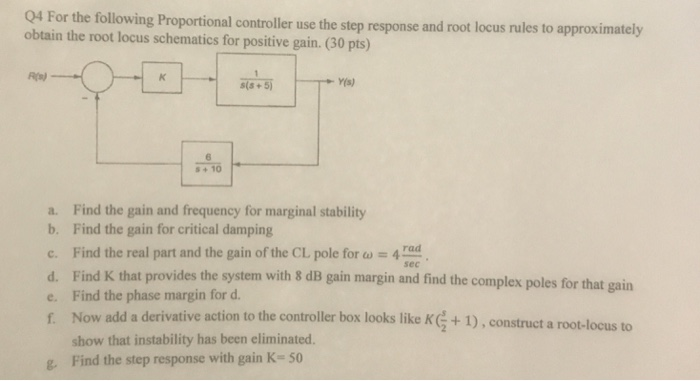Q4 For the following Proportional controller use the step response and root locus rules obtain the root locus schematics for positive gain. (30 pts) Rs) ー10 a. Find the gain and frequency for marginal stability b. Find the gain for critical damping c. Find the real part and the gain ofthe CL pole for a =4 d. Find K that provides the system with 8 dB gain margin and find the complex poles for that gain e. Find the phase...

• ### root locus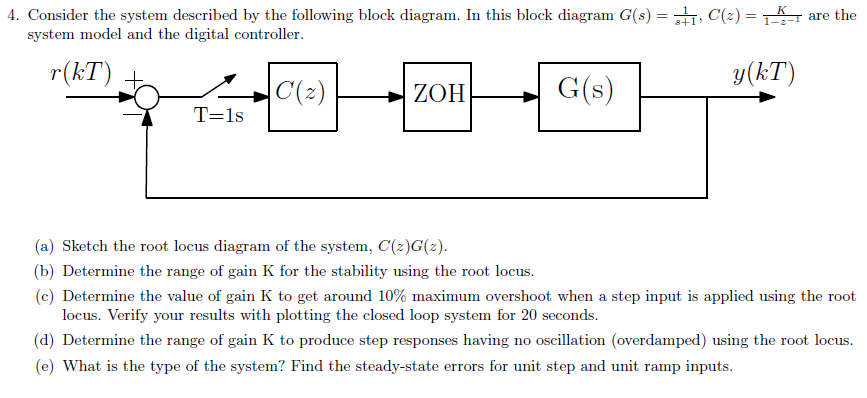4. Consider the system described by the following block diagram. In this block diagram $$G(s)=\frac{1}{s+1}, C(z)=\frac{K}{1-z^{-}}$$ are the system model and the digital controller. (a) Sketch the root locus diagram of the system, $$C(z) G(z)$$. (b) Determine the range of gain $$K$$ for the stability using the root locus. (c) Determine the value of gain $$\mathrm{K}$$ to get around $$10 \%$$ maximum overshoot when a step input is applied using the root locus. Verify your results with plotting the closed...

• ### root locus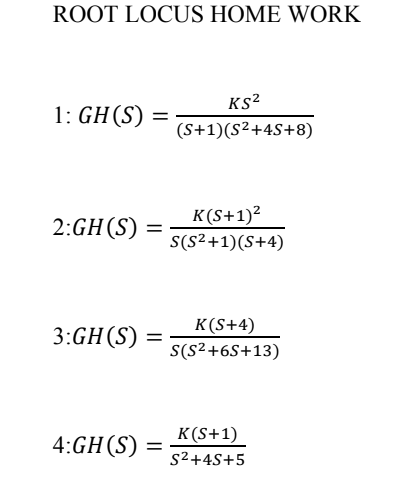• ### Design a phase lead controller using the Root Locus Method for the system described by G(s)....

Design a phase lead controller using the Root Locus Method for the system described by G(s). Ensure that there is a reduction of MORE than 40% of the original settling time, additionally, enure that an overshoot of 12% is not exceeded. G(s)=1/(s3+13s2+32s+20)

• ### Root locus help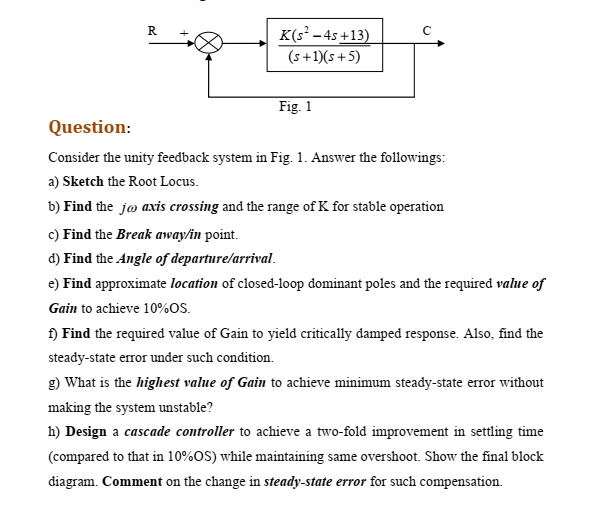• ### Lectures 15-18: Root-locus method 5.1 Sketch the root locus for a unity feedback system with the ...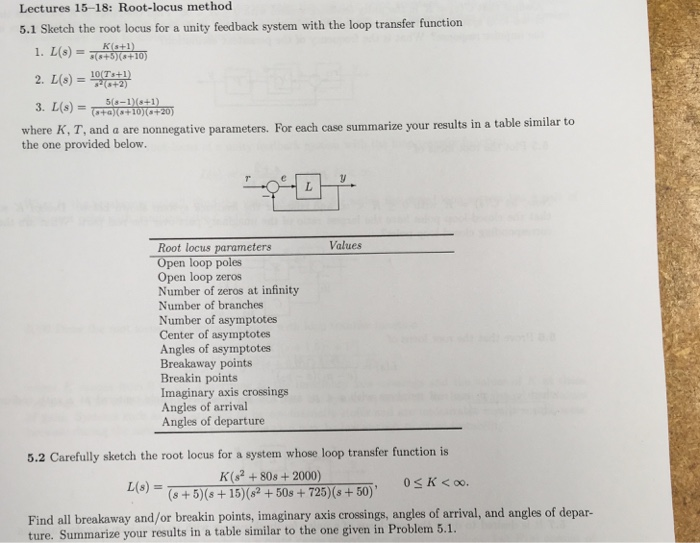help on #5.2 L(s) is loop transfer function 1+L(s) = 0 lecture notes: Lectures 15-18: Root-locus method 5.1 Sketch the root locus for a unity feedback system with the loop transfer function (8+5(+10) .2 +10+20 where K, T, and a are nonnegative parameters. For each case summarize your results in a table similar to the one provided below. Root locus parameters Open loop poles Open loop zeros Number of zeros at infinity Number of branches Number of asymptotes Center of...

• ### design this compensator using root locus? note: answer using root locus 1- Consider a system with the following...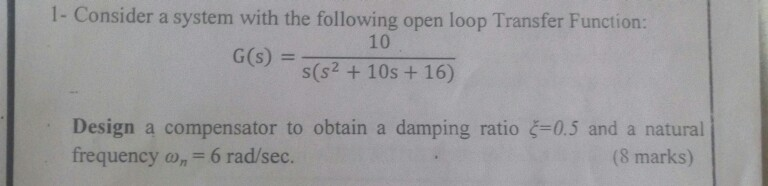design this compensator using root locus? note: answer using root locus 1- Consider a system with the following open loop Transfer Function: G(s)--10 s(s2 + 10s + 16) Design a compensator to obtain a damping ratio-0.5 and a natural frequency n6 rad/sec. (8 marks) We were unable to transcribe this image 1- Consider a system with the following open loop Transfer Function: G(s)--10 s(s2 + 10s + 16) Design a compensator to obtain a damping ratio-0.5 and a natural frequency...

Free Homework App